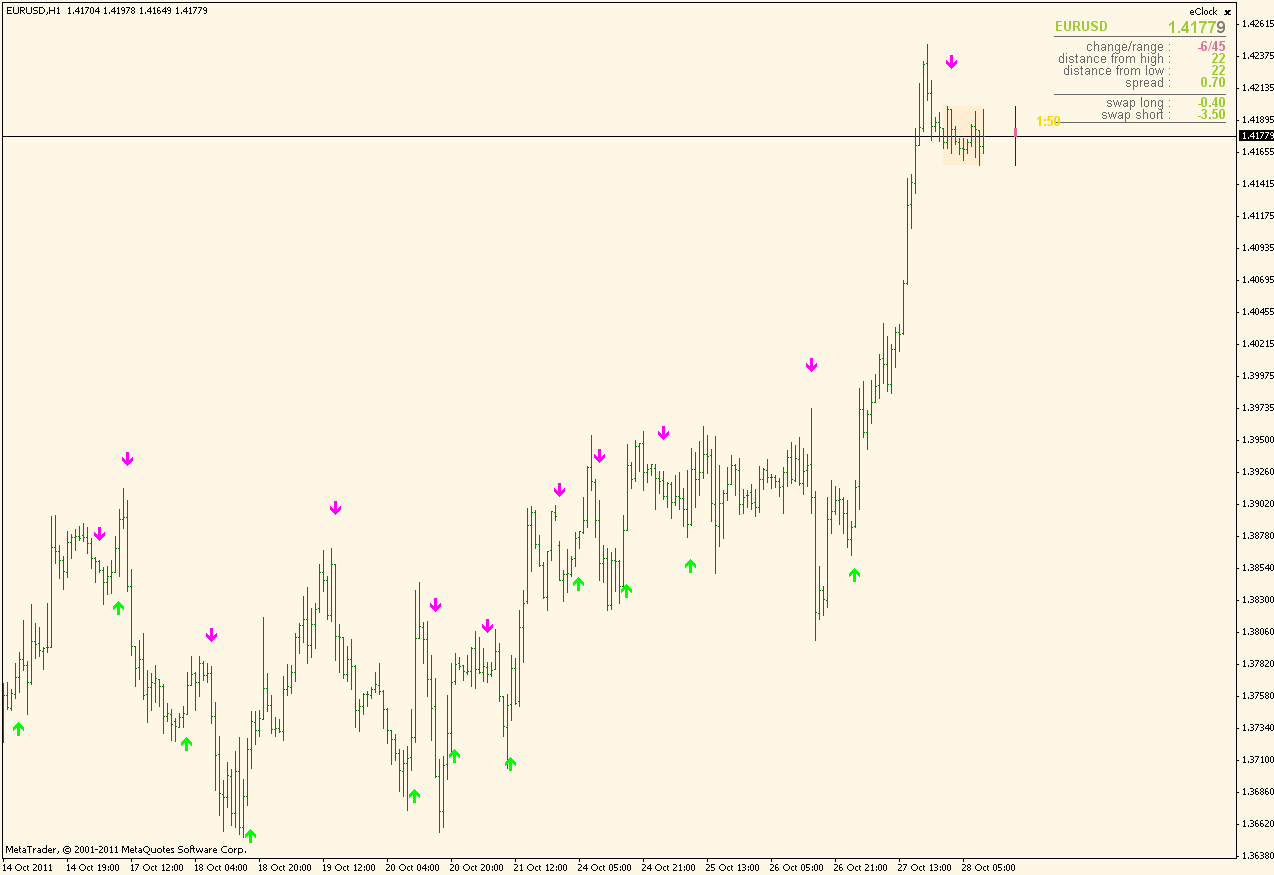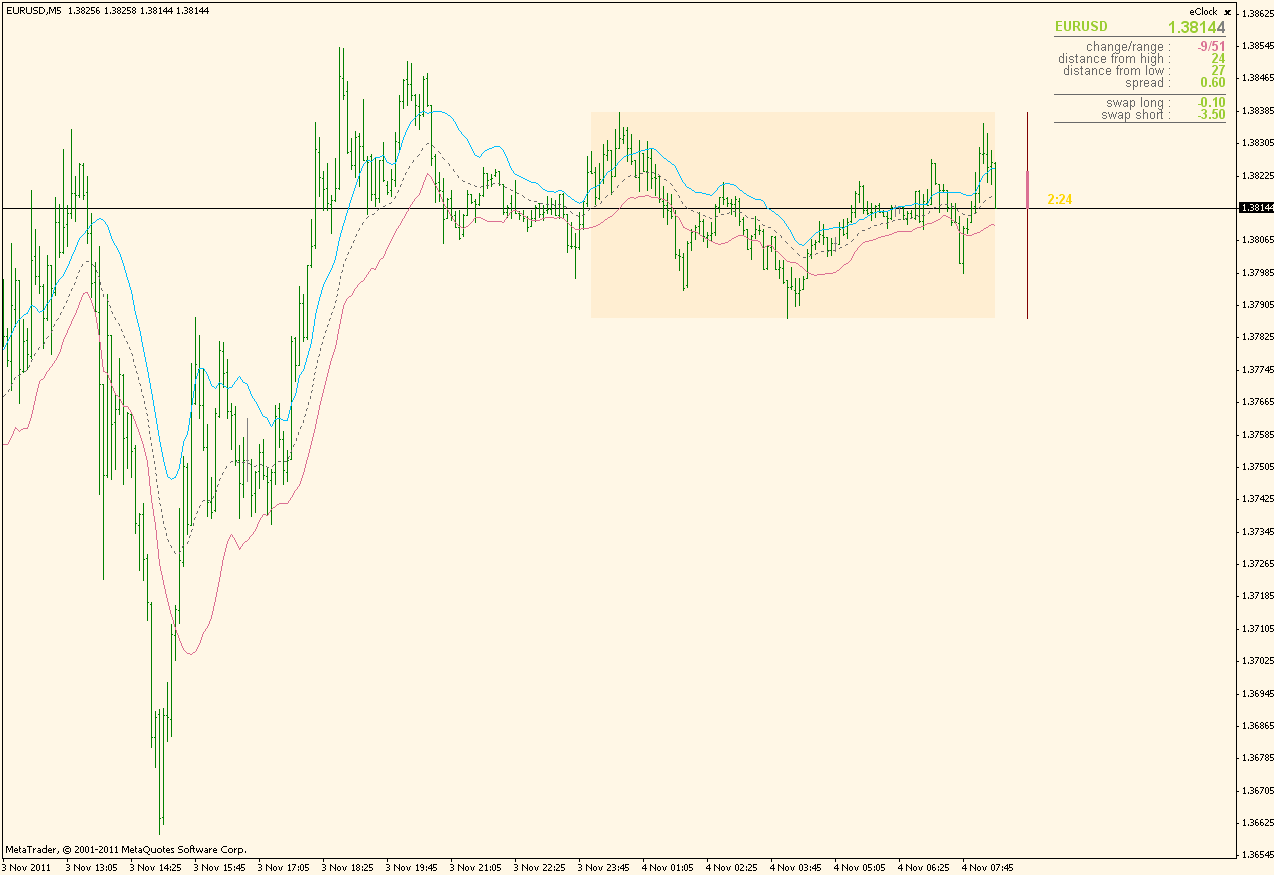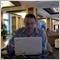# Trend indicators - page 209

gorangel:
@Doody

@xx3xxx

No problem.

its ok buddy... i just got confused cuz i have added an indicator just b4 that and u came up sayin tht it repaints lol...91

Ohhh, Sorry Doody! I just found your indicator (trendisgnal)9

gorangel:
Ohhh, Sorry Doody! I just found your indicator (trendisgnal)

its ok gorangel...

i had sum doubts because sum1 else had told me b4 that it repaints... but i have been checking it for a long while now n i dont think it does repaint...

if u got time, u can check it...

cheers155797

doody

You are right. The indicator you posted does not repaint.

Here is the same thing but written differently (simpler code, no restriction in bars (it works for the whole chart now)) I think it will be clearer what does it do (from the code) now and it is more suitable for further working on now.PS: kept all the logic same as in the original

regards

doody:
its ok gorangel...

i had sum doubts because sum1 else had told me b4 that it repaints... but i have been checking it for a long while now n i dont think it does repaint...

if u got time, u can check it...

cheers
Files:49

Kirshenbaum Bands

Hi all,

I've searched the internet for the indicator, but I found nothing. Can someone make the indicator?

Description:

Kirshenbaum bands are channel lines drawn around an exponential moving average (see Exponential Moving Average). The channel width is a multiple of the “standard error” from a linear regression of a past N days (see Linear Regression, and the EMA is smoothed using the same N days.

Kirshenbaum bands are similar to Bollinger bands (see Bollinger Bands), but with a linear regression standard error (stderr) instead of a standard deviation (stddev, see Standard Deviation). The difference is that stddev takes no account of a trend, so the Bollinger channel widens when a trend is in progress. But stderr is based on deviation from a fitted sloping line, so if prices are making steady progress up or down the channel width remains small.

The standard error values (ie. the channel width) can be viewed directly as an indicator too as “Linear Regression Stderr” (see Linear Regression).

or :

KBA-C Kirshenbaum Bands Paul Kirshenbaum, a money manager and mathematician with a Ph.D. in economics from NYU, submitted this rather unique trading band which is “de-trended.” Kirshenbaum Bands are similar to Bollinger Bands (see BOL-C) in that they measure market volatility. However, rather than use standard deviation of a moving average for band width, they use the standard error of linear regression lines of the close. This has the effect of measuring volatility around the current trend, instead of measuring volatility for changes in trend. Construction: Kirshenbaum Bands are constructed as follows:

Calculate a P-Period Exponential Moving Average of the data based on the close.

Then, for each bar, calculate the L-Period linear regression line, using today’s close as the endpoint of the line. (Note: The term “linear regression” is the same as a “least squares” or “best fit” line in some textbooks.)

Calculate d1, d2, d3, .. dL as the distance from the line to the close on each bar which was used to derive the line. That is, di = Distance from Regression Line to each bar’s close.

Calculate the average of the squared errors:

AE = (d12 + d22 + d32 + .. + dN2) / L

Standard Error (Se) is the square root of this value:

Se = square root of AE

Then, if N = Number of Standard Errors, band width is:

BW = N * SE

Add and subtract the band width from the Exponential Moving Average to arrive at today’s value for the upper and lower bands.

Parameters: Periods (P): The period used in the Exponential Moving Average calculation. Linear Regression Periods (L): The period used in constructing the lines for Linear Regression. Deviations (N): Number of deviations used. That is, the Standard Error value can be multiplied by a factor to expand the bands. Mr. Kirshenbaum recommends a value of 1.75.

Kirshenbaum Bands yield excellent volatility bands. Compare this systems with the Bollinger Bands. Use Kirshenbaum bands to measure volatility around a trend, and Bollinger Bands to measure changes in trend.

I have found the following code : Kirshenbaum Band Lower (KBL)

' Use for the underlying indicator values, indexed by bar index.

Define values() As Number = IndicatorValues(_indicatorKey, barIndex, length + 2 * MathMax(_periods1, _periods2))

' Use for the sum of X times Y, the sum of X, the sum of Y, the sum of X^2

Define sumXY,sumX,sumY,sumXPower As Number

' Use for the EMA smoothing factor.

Define smoothFactor As Number = 2 / (_periods1 + 1)

' Use for the EMA calculation.

Define EMA As Number

' Use for the linear regression calculation.

Define LR As Number

' Use for the average of the squared errors.

Define averageError As Number

' Use for the calculated indicator script values, indexed by bar index.

Define results(length - 1) As Number

' Calculate the indicator script values for the specified bar range.

For i As Integer = length - 1 To 0 Step -1

EMA = 0

' Calculate the indicator script value for the current bar.

For j As Integer = i + _periods1 - 1 To i Step -1

If (EMA 0) Then

EMA = (1 - smoothFactor) * EMA + smoothFactor * values(j)

Else

EMA = values(j)

End If

Next

averageError = 0

' Calculate the linear regression and the average of the squared errors.

For j As Integer = i + _periods2 - 1 To i Step -1

LR = sumXY = sumX = sumY = sumXPower = 0

For k As Integer = j + _periods2 - 1 To j Step -1

sumXY += k * values(k)

sumX += k

sumY += values(k)

sumXPower += k * k

Next

LR = (sumY - (1 * ((_periods2 * sumXY) - (sumX*sumY)) / (_periods2 * sumXPower - (sumX * sumX))) * sumX) / _periods2

averageError += MathPow(values(j) - LR, 2)

Next

averageError = MathSqrt(averageError / _periods2)

results(i) = EMA - averageError

Next

Return results

Thanks and Regards

derumuro

Files:155797

Kirshenbaum bands ...

This should be the onePS: if you want to compare it to Bollinger bands then set the "Mode" parameter to 0 (simple moving average) since Bollinger bands use simple moving average for middle line (not EMA as Kirshenbaum bands )

regards

derumuro:
Hi all,

I've searched the internet for the indicator, but I found nothing. Can someone make the indicator?

Description:

Kirshenbaum bands are channel lines drawn around an exponential moving average (see Exponential Moving Average). The channel width is a multiple of the “standard error” from a linear regression of a past N days (see Linear Regression, and the EMA is smoothed using the same N days.

Kirshenbaum bands are similar to Bollinger bands (see Bollinger Bands), but with a linear regression standard error (stderr) instead of a standard deviation (stddev, see Standard Deviation). The difference is that stddev takes no account of a trend, so the Bollinger channel widens when a trend is in progress. But stderr is based on deviation from a fitted sloping line, so if prices are making steady progress up or down the channel width remains small.

The standard error values (ie. the channel width) can be viewed directly as an indicator too as “Linear Regression Stderr” (see Linear Regression).

or :

KBA-C Kirshenbaum Bands Paul Kirshenbaum, a money manager and mathematician with a Ph.D. in economics from NYU, submitted this rather unique trading band which is “de-trended.” Kirshenbaum Bands are similar to Bollinger Bands (see BOL-C) in that they measure market volatility. However, rather than use standard deviation of a moving average for band width, they use the standard error of linear regression lines of the close. This has the effect of measuring volatility around the current trend, instead of measuring volatility for changes in trend. Construction: Kirshenbaum Bands are constructed as follows:

Calculate a P-Period Exponential Moving Average of the data based on the close.

Then, for each bar, calculate the L-Period linear regression line, using today’s close as the endpoint of the line. (Note: The term “linear regression” is the same as a “least squares” or “best fit” line in some textbooks.)

Calculate d1, d2, d3, .. dL as the distance from the line to the close on each bar which was used to derive the line. That is, di = Distance from Regression Line to each bar’s close.

Calculate the average of the squared errors:

AE = (d12 + d22 + d32 + .. + dN2) / L

Standard Error (Se) is the square root of this value:

Se = square root of AE

Then, if N = Number of Standard Errors, band width is:

BW = N * SE

Add and subtract the band width from the Exponential Moving Average to arrive at today’s value for the upper and lower bands.

Parameters: Periods (P): The period used in the Exponential Moving Average calculation. Linear Regression Periods (L): The period used in constructing the lines for Linear Regression. Deviations (N): Number of deviations used. That is, the Standard Error value can be multiplied by a factor to expand the bands. Mr. Kirshenbaum recommends a value of 1.75.

Kirshenbaum Bands yield excellent volatility bands. Compare this systems with the Bollinger Bands. Use Kirshenbaum bands to measure volatility around a trend, and Bollinger Bands to measure changes in trend.

I have found the following code : Kirshenbaum Band Lower (KBL)

' Use for the underlying indicator values, indexed by bar index.

Define values() As Number = IndicatorValues(_indicatorKey, barIndex, length + 2 * MathMax(_periods1, _periods2))

' Use for the sum of X times Y, the sum of X, the sum of Y, the sum of X^2

Define sumXY,sumX,sumY,sumXPower As Number

' Use for the EMA smoothing factor.

Define smoothFactor As Number = 2 / (_periods1 + 1)

' Use for the EMA calculation.

Define EMA As Number

' Use for the linear regression calculation.

Define LR As Number

' Use for the average of the squared errors.

Define averageError As Number

' Use for the calculated indicator script values, indexed by bar index.

Define results(length - 1) As Number

' Calculate the indicator script values for the specified bar range.

For i As Integer = length - 1 To 0 Step -1

EMA = 0

' Calculate the indicator script value for the current bar.

For j As Integer = i + _periods1 - 1 To i Step -1

If (EMA 0) Then

EMA = (1 - smoothFactor) * EMA + smoothFactor * values(j)

Else

EMA = values(j)

End If

Next

averageError = 0

' Calculate the linear regression and the average of the squared errors.

For j As Integer = i + _periods2 - 1 To i Step -1

LR = sumXY = sumX = sumY = sumXPower = 0

For k As Integer = j + _periods2 - 1 To j Step -1

sumXY += k * values(k)

sumX += k

sumY += values(k)

sumXPower += k * k

Next

LR = (sumY - (1 * ((_periods2 * sumXY) - (sumX*sumY)) / (_periods2 * sumXPower - (sumX * sumX))) * sumX) / _periods2

averageError += MathPow(values(j) - LR, 2)

Next

averageError = MathSqrt(averageError / _periods2)

results(i) = EMA - averageError

Next

Return results

Thanks and Regards

derumuro
Files:49

Kirshenbaum Bands

thanks for the indicator. You have made a good job and very fast.

Regards

derumuro998

RSI_TripleHull Ind

The RSI_TripleHull Ind is already posted here but here it is again to save looking.

Do you know if there is an alert for this indicator?

Thanks.

Files:Moderator
1137184

[langtitle=fr]hello[/langtitle]

[lang=fr]hello all,

I wanted to ask the indicator mladen is using, the one that draws a square in background, can you tell me which one it is?

Also the one on the upper right which indicates the pips from high and low and so on,

thank you[/lang]